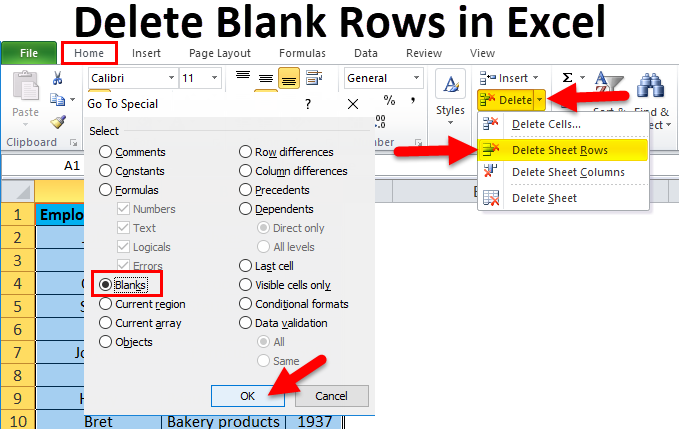# How To Use VBA Delete Blank Cells In Excel

Oct 23, 2020 • edited Oct 24, 2020Below we will look at a program in Excel VBA that deletes blank cells.

Situation:1. First, we declare two variables of type Integer. One named counter and one named i. We initialize the variable counter with value 0.

Dim counter As Integer, i As Integer

counter = 0

2. Next, we check for each cell whether it is empty or not (<> means not equal to). We are using a loop for this. If not empty, we write the value to column B. The counter holds track of the number of cells that have been copied to column B. Each time we copy a value to column B, we increment counter by 1. This piece of the program looks as follows:

``````          For i = 1 To 10

If Cells(i, 1).Value &lt;&gt; &quot;&quot; Then
``````

Cells(counter + 1, 2).Value = Cells(i, 1).Value

counter = counter + 1

End If

Next i

Result so far:3. Finally, we empty Range("A1:A10"), copy the values of column B to column A, and empty Range("B1:B10").

Range("A1:A10").Value = ""

Range("A1:A10").Value = Range("B1:B10").Value

Range("B1:B10") = ""

Result:#Tutorial#How To#VBA#If Then Statement

How To Use VBA Delay a Macro In Excel

How To Use VBA Dependent Combo Boxes In Excel